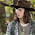Saturday, July 29, 2017

Reinforcing bars should be well anchored so that the bond forces are safely transmitted to the concrete to avoid longitudinal cracking or spalling. Transverse reinforcement shall be provided if necessary. Types of anchorage are shown in the figure below (Figure 8.1 EC2).

For bent bars, the basic tension anchorage length is measured along the centreline of the bar from the section in question to the end of the bar, where:

lbd = α1 α2 α3 α4 α5 lb,req ≥ lb,min ------------ (1)

where;
lb,min is the minimum anchorage length taken as follows:
In tension, the greatest of 0.3lb,rqd or 10 ϕ or 100mm
In compression, the greatest of 0.6lb,rqd or 10 ϕ or 100mm

lb,rqd is the basic anchorage length given by;
lb,rqd = (ϕ/4) σsd/fbd -------------- (2)

Where;
σsd = The design strength in the bar (take 0.87fyk)
fbd = The design ultimate bond stress (for ribbed bars = 2.25η1 η2 fctd)
fctd = Design concrete tensile strength fctd = 0.21fck(2/3) for fck ≤ 50 N/mm2
η1 is a coefficient related to the quality of the bond condition and the position of the bar during concreting
η1 = 1.0 when ‘good’ conditions are obtained and
η1 = 0.7 for all other cases and for bars in structural elements built with slip-forms, unless it can be shown that ‘good’ bond conditions exist
η2 is related to the bar diameter:
η2 = 1.0 for φ ≤ 32 mm
η2 = (132 - φ)/100 for φ > 32 mm

α1 is for the effect of the form of the bars assuming adequate cover.
α2 is for the effect of concrete minimum cover.

α3 is for the effect of confinement by transverse reinforcement
α4 is for the influence of one or more welded transverse bars ( φt > 0.6φ) along the design anchorage length lbd
α5 is for the effect of the pressure transverse to the plane of splitting along the design anchorage length.

The values of these coefficients can be adequately obtained by following the Table below;

CALCULATION OF LAP LENGTH
The design lap length of reinforcements is given by;
l0 = α1 α2 α3 α5 α6 lb,rqd ≥ l0,min --------- (3)
l0,min = max{0.3α6 lb,rqd; 15ϕ; 200}

α6 = √(ρ1/25) but between 1.0 and 1.5
where ρ1 is the % of reinforcement lapped within 0.65l0 from the centre of the lap

Values of α1, α2, α3 and α5 may be taken as for the calculation of anchorage length but for the calculation of α3, ΣAst,min should be taken as 1.0As(σsd/fyd), with As = area of one lapped bar.

SOLVED EXAMPLE FOR ANCHORAGE LENGTH
Calculate the design tension anchorage length of X16mm bar (fyk = 460 N/mm2, concrete cover = 35mm, Concrete cylinder strength fck = 25 N/mm2) for;

(a) When it is a straight bar
(b) When it is bent into any other shape
Assume good bond conditions

Solution
lbd = α1 α2 α3 α4 α5 lb,req ≥ lb,min
lb,rqd = (ϕ/4) σsd/fbd
fbd =2.25η1 η2 fctd
η1 = 1.0 ‘Good’ bond conditions
η2 = 1.0 bar size ≤ 32

fctd = (αct fctk 0.05)/γc --------------- (3)

where;
fctk 0.05 = characteristic tensile strength of concrete at 28 days = 1.8 N/mm2 (Table 3.1 EC2)
γc = partial (safety) factor for concrete = 1.5
αct = coefficient taking account of long-term effects on the tensile strength, this is an NDP with a recommended value of 1.

fctd = (1.0 × 1.8)/1.5 = 1.2 N/mm2
fbd = 2.25 × 1.0 × 1.0 × 1.2 = 2.7 N/mm2

lb,rqd = (ϕ/4) σsd/fbd
σsd = 0.87 × 460 = 400.2 N/mm2>br/> lb,rqd = (ϕ × 400.2 )/(4 × 2.7) = 37.05ϕ

Therefore;

lbd = α1 α2 α3 α4 α5(37.05ϕ)

(a) For straight bar
α1 = 1.0
α2 = 1.0 – 0.15 (Cd – ϕ)/ ϕ
α2 = 1.0 – 0.15 (35 – 16)/16 = 0.8218
α3 = 1.0 conservative value with K = 0
α4 = 1.0 N/A
α5 = 1.0 conservative value

lbd = 0.8218 × (37.05ϕ) = 30.4ϕ = 30.4 × 16 = 486.4 mm
Say 500mm

(b) For other shape bar
α1 = 1.0 bCd; = 35 is ≤ 3ϕ = 3 × 16 = 48
α2 = 1.0 – 0.15 (Cd – 3ϕ)/ ϕ ≤ 1.0
α2 = 1.0 – 0.15 (35 – 48)/16 = 1.121 ≤ 1.0
α3 = 1.0 conservative value with K = 0
α4 = 1.0 N/A
α5 = 1.0 conservative value
lbd = 1.0 × (37.05ϕ) = 37.05ϕ = 37.05 × 16 = 592 mm
Say 600mm

Compression anchorage1 = α2 = α3 = α4 = α5 = 1.0)
lbd = 37.05ϕ

For poor bond conditions
Anchorage for ‘Poor’ bond conditions = ‘Good’/0.7

Example on calculation of lap length of 4X16mm bars of a column in a multi-storey building
Since the bars are in compression,
α1 α2 α3 α5  = 1.0
As calculated above,  lbd = 37.05ϕ

Let us say that over 50% of reinforcement is lapped within 0.65l0 from the centre of the lap
Hence, we will  take α= 1.5

Lap length therefore = 1.5  × 37.05ϕ = 55.57ϕ = 55.57 × 16 = 889.2mm
Say 900 mm

Thank you so much for visiting. You can like our facebook page on

1.2.